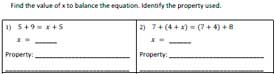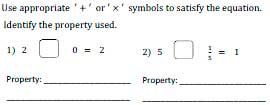# Properties Worksheets

This assortment of printable properties worksheets includes exclusive pages for addition and multiplication properties. It also contains combined worksheets involving both the properties of addition and multiplication. The pdf exercises best suit students of grade 1 through grade 7. Explore some of them for free!

Addition properties worksheets include special focus in each property of addition. Additive inverse and identity worksheets included.

Multiplication Properties Worksheets

Multiplication properties worksheets include practice problems based on commutative, associative, distributive, identity and inverse.

Properties Worksheets: Mixed Review

## Commutative and Associative Properties

Framing the Statement

Use the numbers and form the commutative property statement of addition or multiplication.

Associative Statement

Mixed Review:

Property statement 1

Property statement 2

Find the Missing Numbers

The printable properties worksheets for 3rd grade and 4th grade kids include commutative and associative properties of addition and multiplication. Fill in the missing numbers and find what property is used.

Identifying property 1

Identifying property 2

Solve the Equation

Compare both left and right hand side of the equations to find the value of x and the property used.Solve the Equation 2

## Identity and Inverse

Missing Operators

Identify the property and use the appropriate operator + or x.Identity and Inverse 2

Find the Inverse

Additive inverse of a is -a and multiplicative inverse of a is 1/a. Using these facts and solve the pdf worksheets for 6th grade and 7th grade students.

Inverse Worksheet: Easy

Inverse Worksheet: Moderate

## Properties Worksheets: Revision

Property Chart

The chart displays the properties of addition and multiplication with the separate column for kids to set the own example for each property.Property Chart 2

Underline the Correct Choices

You have list of equations. Underline the equations that best describes the property.

List of Equation 1

List of Equation 2